# GSEB Solutions Class 10 Maths Chapter 14 Statistics Ex 14.2

Gujarat Board GSEB Solutions Class 10 Maths Chapter 14 Statistics Ex 14.2 Textbook Questions and Answers.

## Gujarat Board Textbook Solutions Class 10 Maths Chapter 14 Statistics Ex 14.2

Question 1.
The following table shows the ages of the patients admitted in a hospital for a year.Find the mode and the mean of the data given above. Compare and interpret the two measures of
central tendency.
Solution:
Mode: Here, the maximum frequency is 23 and the class corresponding to this frequency is 35 – 45.
So, the modal class is 35 – 45.
Now, size (h) = 10 lower limit (1) of modal class = 35, frequency (f1) of the modal class = 23, frequency (fi) of class proceeding the modal class = 21, frequency (f2) of class succeeding the modal class = 14.
∴ Mode = l + $$\frac{f_{i}-f_{0}}{2 f_{i}-f_{0}-f_{2}}$$ x h = 35 + $$\frac{23-21}{2 \times 23-21-14}$$ x 10 = 35 + $$\frac {2}{11}$$ x 10 = 35 + $$\frac {20}{11}$$ = 35 + 1.8 (approx.) = 36.8 (approx.)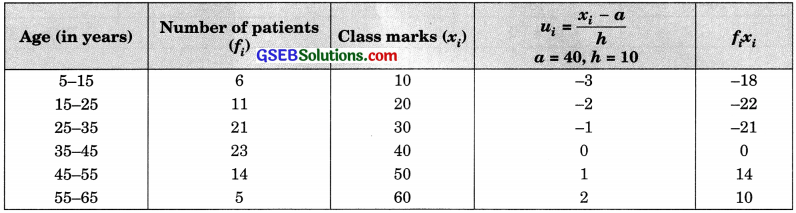Using the step-deviation
$$\bar{x}$$ = a + $$\left(\frac{\Sigma f_{i} u_{i}}{\Sigma f_{i}}\right)$$ x h = 40 + ($$\frac {2}{11}$$) x 10 = 40 – $$\frac {37}{8}$$ = 40 – 4.63 = 35.37years
Interpretation: Maximum number of patients admitted in the hospital are of the age 36.8 years (approx.), while on an average the age of a patient admitted to the hospital is 35.37 years.Question 2.
The following data gives information on the observed lifetimes (in hours) of 225 electrical components.Determine the modal lifetimes of the components.
Solution:
Here, the maximum class frequency is 61, and the class corresponding to this frequency is 60 – 80. So,
the modal class is 60 – 80.
Therefore,
h = 20, l = 60, f1 = 61, f0 = 52, f2 = 38
Mode = l + $$\frac{f_{i}-f_{0}}{2 f_{i}-f_{0}-f_{2}}$$ x h = 60 + $$\frac{61-52}{2 \times 61-52-38}$$ x 20 = 60 + $$\frac{9}{122-90}$$ x 20
= 60 + $$\frac{180}{32}$$ = 60 + $$\frac{45}{8}$$ = 60 + 5.625 = 65.625 hours
Therefore, the modal lifetimes of the component is 65.625 hours.

Question 3.
The following date gives the distribution of total monthly expenditure of 200 families of a village. Find the modal monthly expenditure of the families. Also, find the mean monthly expenditure.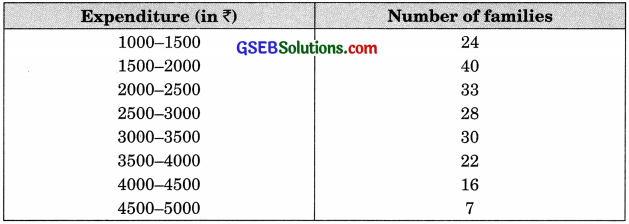Solution:
Mode: Since the maximúm number of families have their total monthly expenditure (in ₹) in the interval 1500 – 2000, the modal class is 1500 – 2000. Therefore,
l = 1500, h = 500, f1 = 40, f0 = 24, f2 = 33
Mode = l + $$\frac{f_{i}-f_{0}}{2 f_{i}-f_{0}-f_{2}}$$ x h = 1500 + $$\left(\frac{40-24}{2 \times 40-24-33}\right)$$ x 500 = 1500 + $$\left(\frac{16}{80-57}\right)$$ x 500 = 1500 + $$\frac{16 x 500}{23}$$ = 1500 + $$\frac{8000}{23}$$ = 1500 + 347.83 = 1847.83
Therefore, the modal monthly expenditure of the families is 1841.83.
Mean: Take a = 3250, h = 500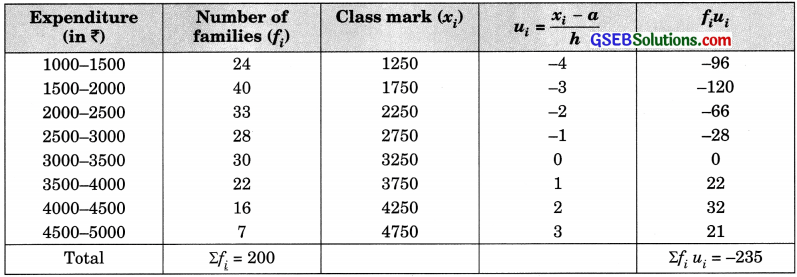Using the step deviation method,
$$\bar{x}$$ = a + $$\left(\frac{\Sigma f_{i} u_{i}}{\Sigma f_{i}}\right)$$ x h = 3250 + $$\frac{- 235 }{200}$$ x 500 = 3250 – $$\frac{235 x 5 }{2}$$ = 3250 – $$\frac{1175 }{2}$$ = 3250 – 587.50 = 2662.50
Hence, the mean monthly expenditure is 2662.50.Question 4.
The following distribution gives the state-wise teacher-student ratio in higher secondary schools of India.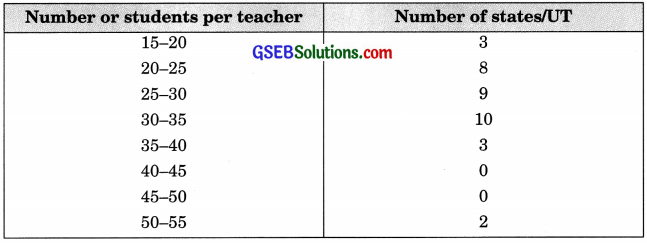Find the mode and mean of this data. Interpret, the two measures
Solution:
Mode: Since the maximum number of states/UT have the number of students per teacher in the interval 30-35, the modal class is 30-35.
Therefore, l = 30, h = 5, f1 = 10, f0= 9, f2 = 3
Mode = l + $$\left(\frac{f_{1}-f_{0}}{2 f_{1}-f_{0}-f_{2}}\right)$$ x h = 30 + $$\left(\frac{10-9}{2 \times 10-9-3}\right)$$ x 5 = 30 + $$\frac{5}{8}$$ = 30 + 0.6 = 30.6
Hence, the mode of the given data is 30.6.
Mean: Take a = 37.5 and h = 5.Using the step deviation method
$$\bar{x}$$ = a + $$\left(\frac{\Sigma f_{i} u_{i}}{\Sigma f_{i}}\right)$$ x h = 37.5 + $$\frac{- 58 }{35}$$ x 5 = 37.5 – 8.3 = 29.2
Interpretation: Most states/UT have a student teacher ratio is 30.6 and on an average this ratio is 29.2.Question 5.
The given distribution shows the number of runs scored by some top batsmen of the world in one-day international cricket matches.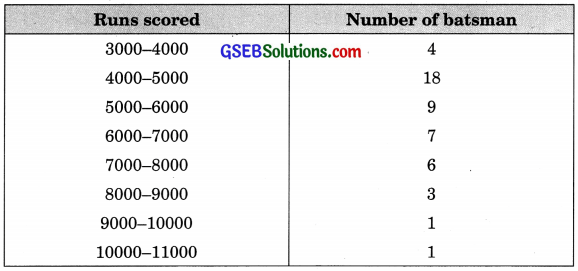Find the mode of the data. (CBSE 2016)
Solution:
Since the maximum number of batsman have their runs scored in the interval 4000-5000, the modal class is 4000-5000.
Therefore, l = 4000, h = 1000, f1 = 18, f0 = 4, f2 = 9
∴ Mode = l + $$\left(\frac{f_{1}-f_{0}}{2 f_{1}-f_{0}-f_{2}}\right)$$ x h = 4000 + $$\left(\frac{18-4}{2 \times 18-4-9}\right)$$ x 1000 = 4000 + 14000 = 4000 + 608.7 = 4608.7
Hence, the mode of the data is 4608.7 runs.Question 6.
A student noted in the number of cars passing through a spot on a road for 100 periods each of 3 minutes and summarised it in the table given below. Find the mode of the data: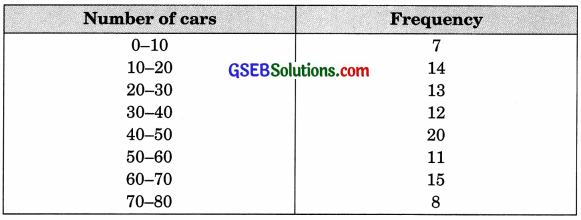Solution:
Here the maximum frequency is 20, and the class corresponding to this frequency is 40-50. So, the modal class is 40-50.
Therefore, l = 40, h = 10, f1 = 20, f0 = 12, f2 = 11
∴ Mode = l + $$\left(\frac{f_{1}-f_{0}}{2 f_{1}-f_{0}-f_{2}}\right)$$ x h = 40 + $$\left(\frac{20-12}{2 \times 20-12-11}\right)$$ x 10 = 40 + $$\frac{80}{17}$$ = 40 + 4.7 = 44.7
Hence, the mode of the data is 44.7 cars.